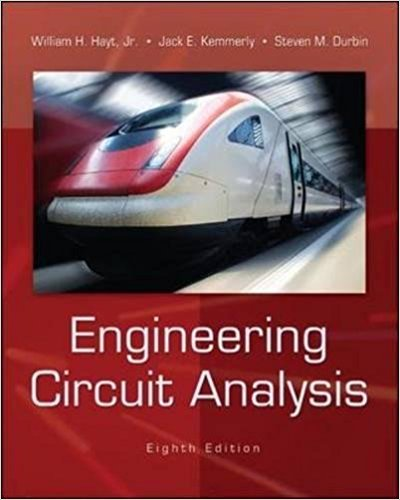×
Get Full Access to Engineering Circuit Analysis - 8 Edition - Chapter 7.1 - Problem 7.2
Get Full Access to Engineering Circuit Analysis - 8 Edition - Chapter 7.1 - Problem 7.2

×

# Determine the current through a 100 pF capacitor if its voltage as a function of time isISBN: 9780073529578 304

## Solution for problem 7.2 Chapter 7.1

Engineering Circuit Analysis | 8th Edition

• Textbook Solutions
• 2901 Step-by-step solutions solved by professors and subject experts
• Get 24/7 help from StudySoup virtual teaching assistantsEngineering Circuit Analysis | 8th Edition

4 5 1 296 Reviews
25
3
Problem 7.2

Determine the current through a 100 pF capacitor if its voltage as a function of time is given by Fig. 7.6.

Step-by-Step Solution:
Step 1 of 3

BJT Amplifiers ▯ 1 ▯= = ▯ 26 ▯ = = 2.6Ω ▯ = ▯= 100Ω ▯ ▯ ▯▯▯...

Step 2 of 3

Step 3 of 3

##### ISBN: 9780073529578

Unlock Textbook Solution